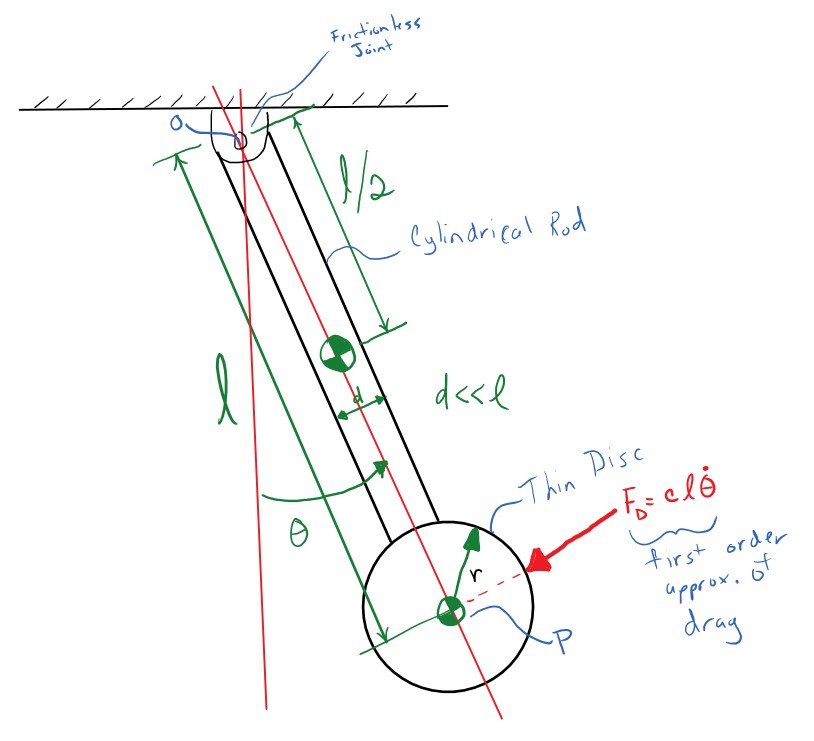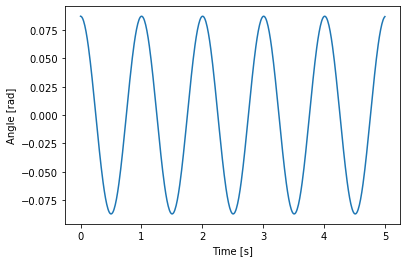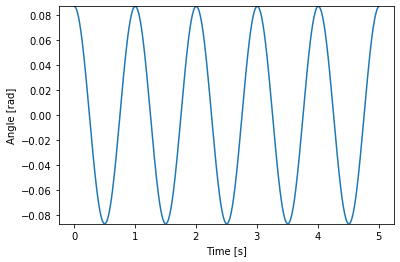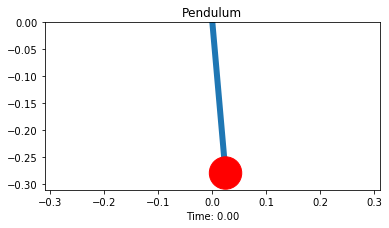# Clock Pendulum with Air Drag Damping¶

This notebook introduces the third fundamental characteristic of vibration: energy dissipation through damping. A compound pendulum system representing a clock pendulum is implemented that allows students to vary the damping parameters and visualize the three regimes of linear damping.

After the completion of this assignment students will be able to:

• understand the concept of damped natural frequency and its relationship to mass/inertia, stiffness, and damping
• state the three fundamental characteristics that make a system vibrate
• compute the free response of a linear system with viscous-damping in all three damping regimes
• identify critically damped, underdamped, and overdamped behavior
• determine whether a linear system is over/under/critically damped given its dynamic properties
• understand the difference between underdamping, overdamping, and crticial damping

## Introduction¶

Many clocks use a pendulum to keep time. Pendulum's have a very constant oscillation period and if designed with quality components take very little energy to run. There is a downside to pendulums though. Any friction in the pendulum's pivot joint or any air drag on the pendulum itself will cause the pendulum to slow stop oscillating and this energy dissaption will affect the period of oscillation. resonance includes a simple clock pendulum that represents a clock pendulum that looks like:Import the pendulum as so:

In :
from resonance.linear_systems import ClockPendulumSystem

In :
sys = ClockPendulumSystem()


Check out its constants. Note that the viscous_damping term is $c$ in the above diagram, i.e. the linear velocity multiplicative factor of the torque due to air drag. Drag is assumed to only act on the bob.

In :
sys.constants

Out:
{'bob_mass': 0.1,
'rod_mass': 0.1,
'rod_length': 0.2799,
'viscous_damping': 0.0,
'acc_due_to_gravity': 9.81}

And the coordinates and speeds:

In :
sys.coordinates

Out:
{'angle': 0.0}
In :
sys.speeds

Out:
{'angle_vel': 0.0}

The system can be simulated as usual if the coordinates or speeds are set to some initial value.

In :
import numpy as np

In :
sys.coordinates['angle'] = np.deg2rad(5.0)

In :
traj = sys.free_response(5.0)


And a plot can be shown:

In :
import matplotlib.pyplot as plt

In :
%matplotlib inline

In :
fig, ax = plt.subplots(1, 1)
ax.plot(traj.index, traj.angle)
ax.set_xlabel('Time [s]');The above simulation shows that we get a sinusoid oscillation with a period of about 1 second, which is good.

Exercise

Creative an interactive plot of the angle trajectory with a slider for the viscous_damping coefficient. The slider shoudl go from 0.0 to 5.0 with step of 0.1. The code should follow this pattern, as before:

fig, ax = plt.subplots(1, 1)

sim_line = ax.plot(traj.index, traj.angle)
ax.set_ylim((-sys.coordinates['angle'], sys.coordinates['angle']))

ax.set_xlabel('Time [s]')

def plot_trajectory(viscous_damping=0.0):
# fill out this function so that the plot will update with a slider.
# 1) update the system's viscous_damping constant
# 2) simulate the free response with free_response()
# 3) update sim_line's data with set_data(x, y)

plot_trajectory()

In :
fig, ax = plt.subplots(1, 1)

sim_line = ax.plot(traj.index, traj.angle)
ax.set_ylim((-sys.coordinates['angle'], sys.coordinates['angle']))

ax.set_xlabel('Time [s]')

def plot_trajectory(viscous_damping=0.0):
sys.constants['viscous_damping'] = viscous_damping
traj = sys.free_response(5.0)
sim_line.set_data(traj.index, traj.angle)

plot_trajectory()In :
# write your answer here

In :
from ipywidgets import interact

In :
widget = interact(plot_trajectory, viscous_damping=(0.0, 5.0, 0.1));


# Visualize the Motion¶

In :
sys.constants['viscous_damping'] = 0.2
frames_per_second = 30
traj = sys.free_response(5.0, sample_rate=frames_per_second)

In :
ani = sys.animate_configuration(fps=frames_per_second, repeat=False)  # sample time in millisecondsThis is another option for animating that gives a set of play buttons and options.

In :
from IPython.display import HTML
HTML(ani.to_jshtml(fps=frames_per_second))

Out: